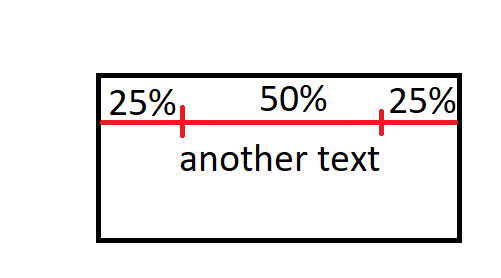# QLabel with resizedText

• Hi,

I would like to have a subclass of QLabel, where the text's width will have a 50% of QLabel's width:my resizeEvent:

``````int textWidth = fontMetrics().horizontalAdvance(text());
``````

And here is the problem: I know that I have to use create QFont variable, setPixelSize() and set this font in QLabel like this: setFont(font).
But how can I calculate textWidth to setPixelSize?

• @TomNow99

• But how can I calculate textWidth to setPixelSize?

Normally you would simply reduce point size until your text fits the area.

something like this. all credits to @SebastienL

``````void scalePainterFontSizeToFit(QPainter &painter, QFont &r_font, float _heightToFitIn)
{
float oldFontSize, newFontSize, oldHeight;

// Init
oldFontSize=r_font.pointSizeF();

// Loop
for (int i=0 ; i<3 ; i++)
{
oldHeight = painter.fontMetrics().boundingRect('D').height();
newFontSize = (_heightToFitIn / oldHeight) * oldFontSize;
r_font.setPointSizeF(newFontSize);
painter.setFont(r_font);
oldFontSize = newFontSize;
//qDebug() << "OldFontSize=" << oldFontSize << "HtoFitIn=" << _heightToFitIn << "  fontHeight=" << oldHeight << "  newFontSize=" << newFontSize;
}

// End
r_font.setPointSizeF(newFontSize);
painter.setFont(r_font);
}
``````

Disclaimer. Not tested how well this code works. Its was more for the concept overall.